# Multiplication With the Stamp Game

Multiplication With the Stamp Game
Math - Arithmetical Operations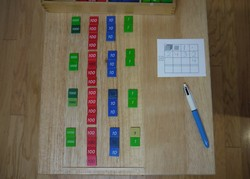LevelPrimary
Age5+
MaterialsStamp Game set
math problem paper
regular pencil
green pencil
blue pencil
red pencil
divider (string, stick, or ruler)

This activity teaches the child to do multiplication at a higher level of abstraction than with the Golden Beads.

## Presentations

1. Take the materials to a table. (A very smooth mat might also be acceptable.)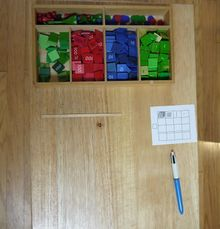2. Select a problem and write it on the paper. In this case: 2432 × 4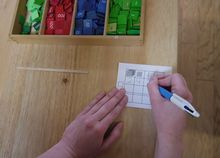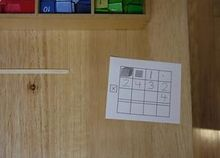3. Lay out the number four times, moving the divider down each time.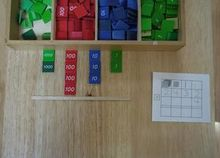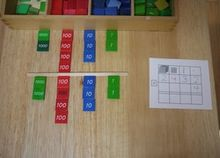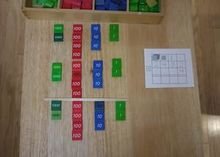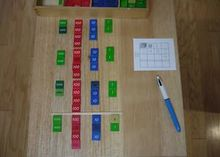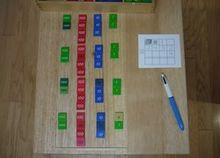4. Slide the stamps in each column together.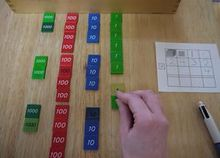5. Then count and record the units (exchanging if needed).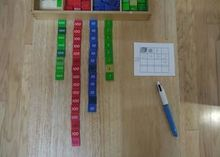6. Count the tens (exchanging for a hundred if needed.)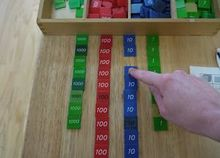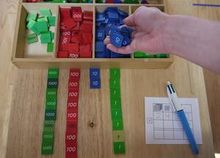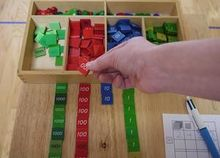7. Record the tens left on the paper.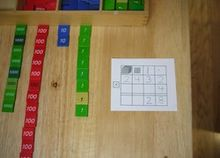8. Then count and record the hundreds (exchanging if needed).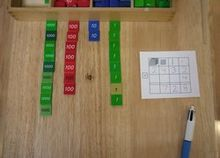9. Then count and record the thousands (exchanging if needed).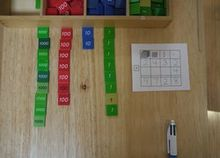## Material

Prices and links last checked: 14 May 2020

Absorbent Montessori \$47.00
Affordable Montessori \$33.00
Alison's \$36.75
Bruins \$89.00
E & O \$48.00
IFIT \$28.00